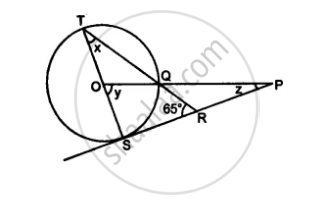Share

# In the Figure Given Below, O is the Center of the Circle of the Circle and Sp is a Tangent. If ∠Srt=65°, Find the Value of X, Y and Z. - Mathematics

Course

#### Question

In the figure given below, O is the center of the circle of the circle and SP is a tangent. if ∠SRT=65°, find the value of x, y and Z.#### Solution

TS ⊥ SP,

⇒ ∠TSR=90°

"In" ΔTSR,

∠TSR+∠TRS+∠RTS=180°

⇒90°+65°+x=180°

⇒x=180°-90°-65°

⇒x=25°

"Now", y=2x  .............("Angle subtended at the center is double that of the angle subtended by the arc at the same centre")

⇒y=2xx25°

⇒y=50°

"In" Δ OSP,

∠OSP+∠SPO+∠POS-=180°

⇒90°+z+50°=180°

⇒z=180°-140°

⇒z=40°

"Hence", x=25°,y=50° and Z=40°

Is there an error in this question or solution?